Star

Calculate the content of the shaded part of square with side 28 cm.

Result

S =  168.248 cm2

Solution:Leave us a comment of example and its solution (i.e. if it is still somewhat unclear...):Be the first to comment!Next similar examples:

1. Annulus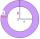Two concentric circles form an annulus of width 10 cm. The radius of the smaller circle is 20 cm. Calculate the content area of annulus.
2. AnnulusThe radius of the larger circle is 8cm, the radius of smaller is 5cm. Calculate the contents of the annulus.
3. CableCable consists of 8 strands, each strand consists of 12 wires with diameter d = 0.5 mm. Calculate the cross-section of the cable.
4. 22/7 circleCalculate approximately area of a circle with radius 20 cm. When calculating π use 22/7.
5. Annulus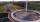Calculate the area of two circles annulus k1 (S, 3 cm) and k2 (S, 5 cm).
6. Circle - easy 2The circle has a radius 6 cm. Calculate:
7. Circle - simpleCalculate the area of a circle in dm2, if its circumference is 31.4 cm.
8. 6-gon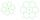Perimeter of regular hexagon is 113. Calculate its circumradius (radius of circumscribed circle).
9. Tripled squareIf you tripled the length of the sides of the square ABCD you increases its content by 200 cm2. How long is the side of the square ABCD?
10. 3x square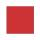Side length of the square is 54 cm. How many times increases the content area of square if the length of side increase three times?
11. CD disc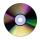A compact disc has a diameter of 11.8 cm. What is the surface area of the disc in square centimeters?
12. Perimeter of squareThe square has a circumference 17cm. What is its area?
13. Square and circle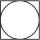Into square is inscribed circle with diameter 10 cm.What is difference between circumference square and circle?
14. SidewalkThe city park is a circular bed of flowers with a diameter of 8 meters, around it the whole length is 1 meter wide sidewalk . What is the sidewalk area?
15. WellRope with a bucket is fixed on the shaft with the wheel. The shaft has a diameter 50 cm. How many meters will drop bucket when the wheels turn 15 times?
16. Two circlesTwo circles with a radius 4 cm and 3 cm have a center distance 0.5cm. How many common points have these circles?
17. Complementary angles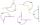Two angles are complementary. If one angle measures 10°, what is the measure of the second angle?